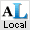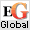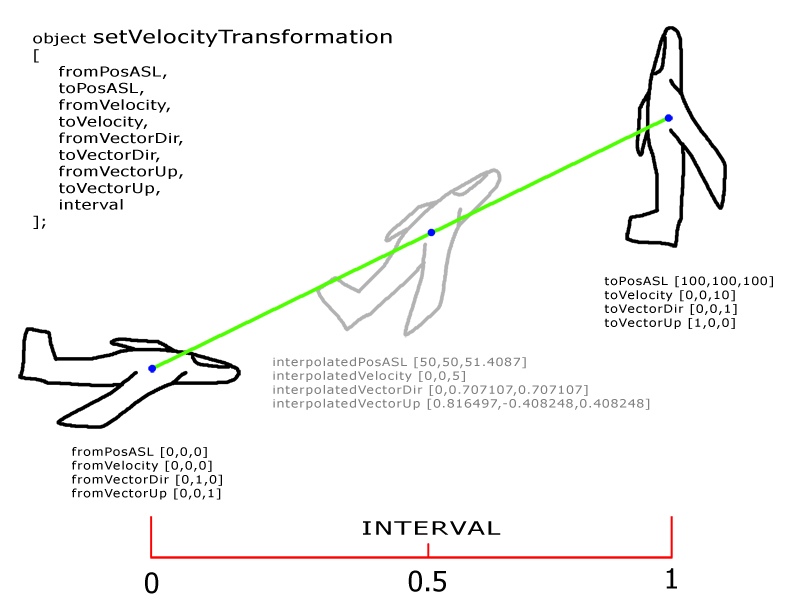# setVelocityTransformationClick on the images for descriptions

### Introduced in

Game:
Arma 2: Operation Arrowhead
Version:
1.51

### Description

Description:
Interpolates and applies PositionASL, velocity, vectorDir and vectorUp to the given object based on the interval value.
When interval is 0 the "fromXXX" values are used for the beginning of the interpolation. When interval is 1, the "toXXX" values are used for the end of interpolation. When interval value is in between, interpolated values are used (see diagram below). The interpolation is linear and along straight line between "from" and "to" positions. If curve is needed, then it should be constructed from multiple straight sections or by dynamically changing value of position params during the interpolation cycle, similar to Quadratic Curve animation (see Example 3).
The velocity param doesn't do much in SP, but in MP, provided the velocity component is set correctly, it helps the engine to figure out what the next position of the moving object should be on other clients.

The actual approximate interpolation formula for this command is: `_vecCurrent = _vecFrom vectorAdd (_vecTo vectorDiff _vecFrom vectorMultiply _interval);`For continuous movement this command should be executed each frame while changing interval value. For example to apply interpolation for 10 seconds one could use the following command to translate 10 seconds into 0...1 interval:

```t1 = time; t2 = time + 10;``` Later inside some on frame event...

`_interval = linearConversion [t1, t2, time, 0, 1];`

In multiplayer, this command should be applied where object is local

### Syntax

Syntax:
object setVelocityTransformation [fromPosASL, toPosASL, fromVelocity, toVelocity, fromVectorDir, toVectorDir, fromVectorUp, toVectorUp, interval]
Parameters:
object: Object - object to be interpolated
[fromPosASL, toPosASL, fromVelocity, toVelocity, fromVectorDir, toVectorDir, fromVectorUp, toVectorUp, interval] : Array
fromPosASL: PositionASL - starting getPosASL of the object
toPosASL: PositionASL - ending getPosASL of the object
fromVelocity: Array - starting velocity of the object
toVelocity: Array - ending velocity of the object
fromVectorDir: Array - starting vectorDir of the object
toVectorDir: Array - ending vectorDir of the object
fromVectorUp: Array - starting vectorUp of the object
toVectorUp: Array - ending vectorUp of the object
interval: Number - interpolation interval (variable value, normally between 0 and 1)
Return Value:
Nothing

### Examples

Example 1:
```_tracker setVelocityTransformation [ _currentPos, _nextPos, _currentVelocity, _nextVelocity, _currentVectorDir, _nextVectorDir, _currentVectorUp, _nextVectorUp, _interval ];```
Example 2:
Bob on imaginary stairway to heaven:```bob = createAgent ["C_man_1", player getRelPos [5, 0], [], 0, "CAN_COLLIDE"]; bob switchMove "ladderciviluploop"; pos1 = getPosASL bob; pos2 = pos1 vectorAdd [0,0,0.75]; bob addEventHandler ["AnimDone", { pos1 = pos2; pos2 = pos2 vectorAdd [0,0,0.75] }]; onEachFrame { if (!alive bob) then { onEachFrame {}; bob switchMove ""; bob removeAllEventHandlers "AnimDone"; }; bob setVelocityTransformation [ pos1, pos2, [0,0,0], [0,0,0], [0,1,0], [0,1,0], [0,0,1], [0,0,1], moveTime bob ]; };```
Example 3:
Advanced trickery with curved movement. The curve is controlled with one control point (controlPointASL), just like quadratic Bézier curve:```disableSerialization; player setDir 0; interval = 0; _disp = findDisplay 46 createDisplay "RscDisplayEmpty"; _ctrl = _disp ctrlCreate ["RscSlider", -1]; _ctrl ctrlSetPosition [safeZoneX + 0.1, 1, safeZoneW - 0.2, 0.1]; _ctrl ctrlSetActiveColor [1,0,0,1]; _ctrl ctrlCommit 0; _ctrl sliderSetPosition 0; _ctrl sliderSetRange [0,1]; _ctrl sliderSetSpeed [0.1,0.5]; _ctrl ctrlAddEventHandler ["SliderPosChanged", {interval = _this select 1}]; ctrlSetFocus _ctrl; box = "Land_VR_Shape_01_cube_1m_F" createVehicle [0,0,0]; controlPointASL = AGLtoASL (player getRelPos [70, -30]) vectorAdd [0, 0, 30]; fromPosASL = AGLtoASL (player getRelPos [10, -45]); toPosASL = AGLtoASL (player getRelPos [10, 45]); fromControlPointOffset = controlPointASL vectorDiff fromPosASL; toControlPointOffset = toPosASL vectorDiff controlPointASL; onEachFrame { hintSilent format ["Interval: %1", interval]; box setVelocityTransformation [ fromPosASL vectorAdd (fromControlPointOffset vectorMultiply interval), controlPointASL vectorAdd (toControlPointOffset vectorMultiply interval), [0,0,0], [0,0,0], [0,1,0], [1,0,0], [0,0,1], [0,1,0], interval ]; };```

(A3 1.24) Generally speaking setVelocityTransformation is more likely a combination of setPosASL, setVectorDirAndUp (or BIS_fnc_setPitchBank) and time multiplier. It can be used as a position tracker with all necessary information collected, copied and then released within one function. Here’s a simple reproduction on how setVelocityTransformation works in game: ``` private ["_dataOld","_dataNext","_capturedData","_obj","_fps","_startTrackingTime","_stepOld","_stepNext","_tracker","_tempTime"]; _stepOld = 0; _tempTime = 0; _stepNext = 1; while {true} do { _capturedData = _capturedData + [[ getPosASL _obj,velocity _obj,vectorDir _obj,vectorUp _obj]]; sleep _fps; _tempTime = _tempTime + _fps; if (_tempTime >= _startTrackingTime) then { _dataOld = _capturedData select _stepOld; _dataNext = _capturedData select _stepNext; _stepOld = _stepOld + 1; _stepNext = if (_stepNext >= (count _capturedData)) then [{_stepOld},{_stepNext + 1}]; _tracker setVelocityTransformation [_dataOld select 0,_dataNext select 0,_dataOld select 1,_dataNext select 1, _dataOld select 2,_dataNext select 2,_dataOld select 3,_dataNext select 3,1]; }; };``` Tracker starts coping the route and stance from the object when time start counting. TimeDiff determines the distance multiply between the current position and the next position.